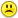+0

# In class we saw that the sum of the entries of row $n$ of Pascal's Triangle is $2^n$. In this problem we investigate the sums of the squares

0
3359
8

$$In class we saw that the sum of the entries of row n of Pascal's Triangle is 2^n. In this problem we investigate the sums of the squares of the entries of row n of Pascal's Triangle. (a) Compute the sums of the squares of Rows 1-4 of Pascal's Triangle. That is, compute:$$\binom10^2 + \binom11^2\binom20^2 + \binom21^2 + \binom22^2\binom30^2 + \binom31^2 + \binom32^2 + \binom33^2\binom40^2 + \binom41^2 + \binom42^2 + \binom43^2 + \binom44^2$$Do these sums appear anywhere else in Pascal's Triangle? (b) Guess at an identity based on your observations from part (a). Your identity should be of the form$$\binom{n}{0}^2 + \binom{n}{1}^2 + \cdots + \binom{n}{n}^2 = \text{ something}.$$(You have to figure out what "something" is.) Test your identity for n=1,2,3,4 using your results from part (a). (c) Prove your identity using a committee-forming argument. (d) Prove your identity using a block-walking argument.$$

Please explain very well for each part in this question.

Apr 24, 2015

#1
+17

Here's part of the question.......

a) The sums are

2 =  C(2,1)

6  = C(4, 2)

20  = C(6, 3)

70= C(8,4)

b) The pattern appears to be

[C(n,0)]^2  + [C(n, 1)]^2  + [C(n, 2)]^2 +.....+ [C(n,n)]^2   = C(2n, n)

Sorry....I don't know the answers to c or d   !!!!Apr 25, 2015

#1
+17

Here's part of the question.......

a) The sums are

2 =  C(2,1)

6  = C(4, 2)

20  = C(6, 3)

70= C(8,4)

b) The pattern appears to be

[C(n,0)]^2  + [C(n, 1)]^2  + [C(n, 2)]^2 +.....+ [C(n,n)]^2   = C(2n, n)

Sorry....I don't know the answers to c or d   !!!!CPhill Apr 25, 2015
#2
-1

Thanks ChrisApr 26, 2015
#3
-1

I am sorry Mellie I don't know either. I don't even know what  committee forming arguements or block walking arguments are :/I'll put it in the wrap, maybe one of the other mathematicians can help youApr 28, 2015
#4
-1

Imagine Pascal's Triangle with lines connecting each term. The ways go from the top to somewhere else is a block-walking proof in this case.

As for the committee-forming proof, we need to form a committee by using

$$\dbinom{n}{k}$$

Apr 24, 2016
#5
+1

Committee forming is just like saying you have $n$ people, and from that $n$ people, you want to choose $k$ people to form a committee or group, so how many different groups can you make? So it's just like $\binom{n}{k}$.

Jul 26, 2016
#6
-1

I still don't get how to do c and d. Can someone please explain or give hints?

Aug 26, 2016
#7
0

Here is a gigantic hint from AoPS Wiki (http://artofproblemsolving.com/wiki/index.php?title=Committee_forming)

Nov 28, 2016
#8
+1

@CPhill: I do not think that your solution to part b adequately explains what "something" is in the equation provided to us. You seem to have put down the same pattern, but not what the pattern is equal to. Please make that clearer. Thanks!

Feb 1, 2017